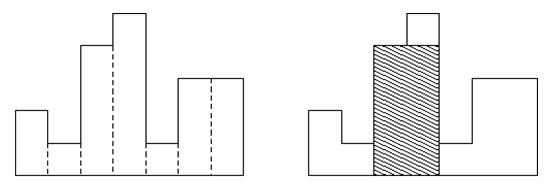Competitions

# Histogram

The histogram is a polygon formed from the sequence of rectangles, aligned on a common baseline. The rectangles are of equal width, but may have different heights. For example, the figure on the left shows the histogram, which consists of rectangles with heights of 2, 1, 4, 5, 1, 3, 3. All rectangles in this figure have a width equal to 1.Usually, histograms are used to represent discrete distributions, for example, the frequency of characters in the texts. Note that the order of the rectangles is very important. Calculate the area of the largest rectangle in the histogram, which is also located on a common baseline. The figure on the right is the shaded figure is equal to the largest aligned rectangle on the image histogram.

#### Input

In the first line of the input file is written the number n (0n106) - number of rectangles of the histogram. This is followed by n integers h1, ..., hn (0hi109). These numbers indicate the height of the rectangle of the histogram from left to right. The width of each rectangle is equal to 1.

#### Output

Display area of the large rectangle in the histogram. Remember that this rectangle should be on a common baseline.

Time limit 1 second
Memory limit 128 MiB
Input example #1
7 2 1 4 5 1 3 3

Output example #1
8

Input example #4
8 2 1 2 0 3 2 2 3

Output example #4
8

Source Отборочный тур Всероссийской командной олимпиады школьников 2009/2010 учебный год